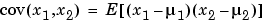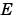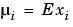MATLAB Function Referencecov

Covariance matrix

Syntax

• ```C = cov(X)
C = cov(x,y)
```

Description

`cov(X)`, if `X` is a vector, returns the variance. For matrices, where each row is an observation, and each column is a variable, `cov(X)` is the covariance matrix. `diag(cov(X))` is a vector of variances for each column, and `sqrt(diag(cov(X)))` is a vector of standard deviations. `cov(X,Y)`, where `X` and `Y` are vectors of equal length, is equivalent to `cov([X(:) Y(:)])`.

`cov(X)` or `cov(X,Y)` normalizes by N-1 where N is the number of observations. This makes `cov(X)` the best unbiased estimate of the covariance matrix if the observations are from a normal distribution.

`cov(X,1)` or `cov(X,Y,1)` normalizes by N and produces the second moment matrix of the observations about their mean. `cov(X,Y,0)` is the same as `cov(X,Y)` and `cov(X,0)` is the same as `cov(X)`.

Remarks

`cov` removes the mean from each column before calculating the result.

The covariance function is defined as

•whereis the mathematical expectation and.

Examples

Consider `A = [-1 1 2 ; -2 3 1 ; 4 0 3]`. To obtain a vector of variances for each column of `A`:

• ````v = diag(cov(A))`'
v =
10.3333    2.3333    1.0000
```

Compare vector `v` with covariance matrix `C`:

• ```C =
10.3333   -4.1667    3.0000
-4.1667    2.3333   -1.5000
3.0000   -1.5000    1.0000
```

The diagonal elements `C(i,i)` represent the variances for the columns of `A`. The off-diagonal elements `C(i,j)` represent the covariances of columns `i` and `j`.

See Also

`corrcoef`, `mean`, `median`, `std`, `var`

`xcorr`, `xcov` in the Signal Processing Toolbox

© 1994-2005 The MathWorks, Inc.